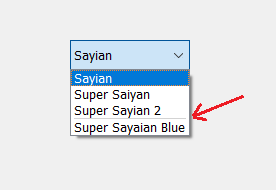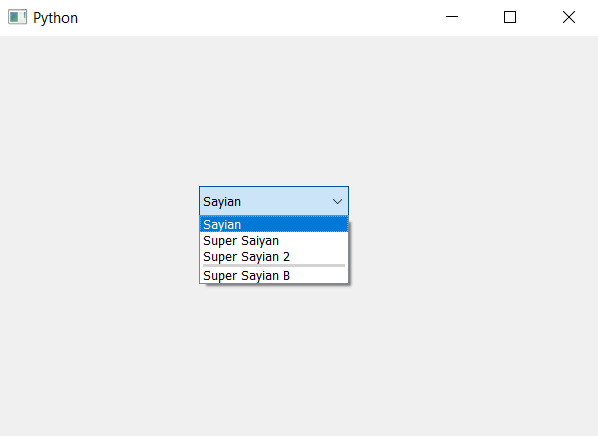# PyQt5 – Increase size of separator in between the item of ComboBox

• Last Updated : 20 Jun, 2021

In this article we will see how we can increase the size of separator at the desired index in the combo box. By default there is no separator. Separator is the black line in between two items, in order to add he separator we use insertSeparator method. Below is representation of how combo box items with a separator looks like –In order to increase the size of separator we have to use the insertSeparator method multiple time with the index value equal to desired index or greater than that this will increase the size of separator of given index.

Syntax :
combo_box.insertSeparator(index)
combo_box.insertSeparator(index)
Argument : It takes integer as argument i.e index
Action performed : It will add separator at the given index and using same statement with same index will increase the size of separator

Note : Index value must be greater or equal to the desired index.
Below is the implementation –

## Python3

 `# importing libraries``from` `PyQt5.QtWidgets ``import` `*``from` `PyQt5 ``import` `QtCore, QtGui``from` `PyQt5.QtGui ``import` `*``from` `PyQt5.QtCore ``import` `*``import` `sys`  `class` `Window(QMainWindow):` `    ``def` `__init__(``self``):``        ``super``().__init__()` `        ``# setting title``        ``self``.setWindowTitle(``"Python "``)` `        ``# setting geometry``        ``self``.setGeometry(``100``, ``100``, ``600``, ``400``)` `        ``# calling method``        ``self``.UiComponents()` `        ``# showing all the widgets``        ``self``.show()` `    ``# method for widgets``    ``def` `UiComponents(``self``):` `        ``# creating a combo box widget``        ``self``.combo_box ``=` `QComboBox(``self``)` `        ``# setting geometry of combo box``        ``self``.combo_box.setGeometry(``200``, ``150``, ``150``, ``30``)` `        ``# geek list``        ``geek_list ``=` `[``"Sayian"``, ``"Super Saiyan"``, ``"Super Sayian 2"``, ``"Super Sayian B"``]` `        ``# adding list of items to combo box``        ``self``.combo_box.addItems(geek_list)` `        ``# index``        ``index ``=` `3` `        ``# adding separator at given index``        ``self``.combo_box.insertSeparator(index)` `        ``# increasing the size of separator``        ``self``.combo_box.insertSeparator(index)``        ``self``.combo_box.insertSeparator(index)` `# create pyqt5 app``App ``=` `QApplication(sys.argv)` `# create the instance of our Window``window ``=` `Window()` `# start the app``sys.exit(App.``exec``())`

Output :My Personal Notes arrow_drop_up